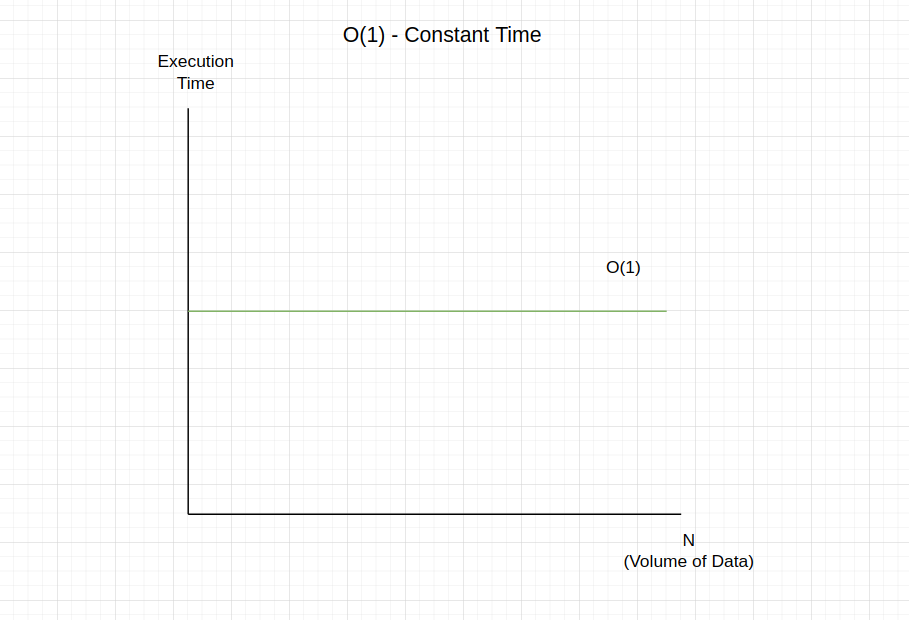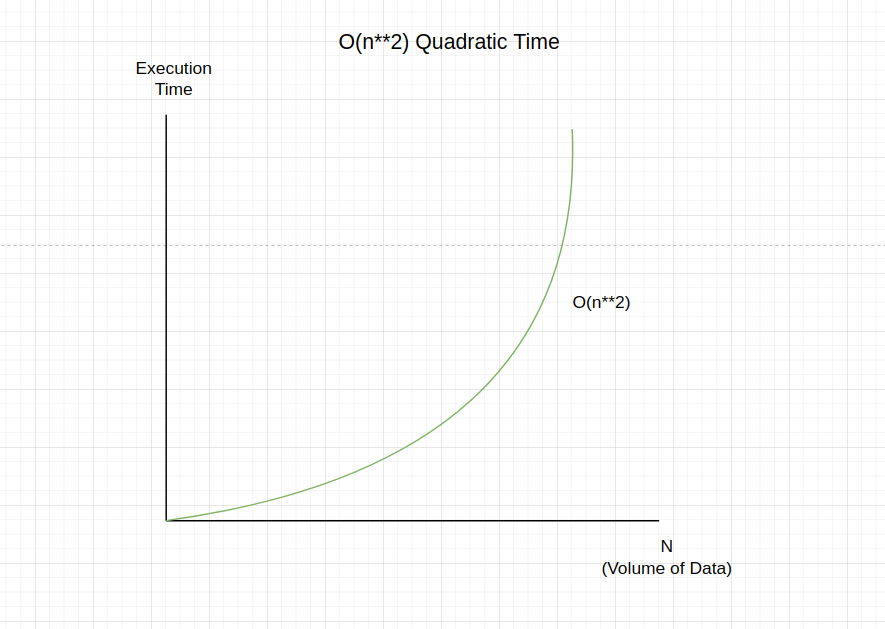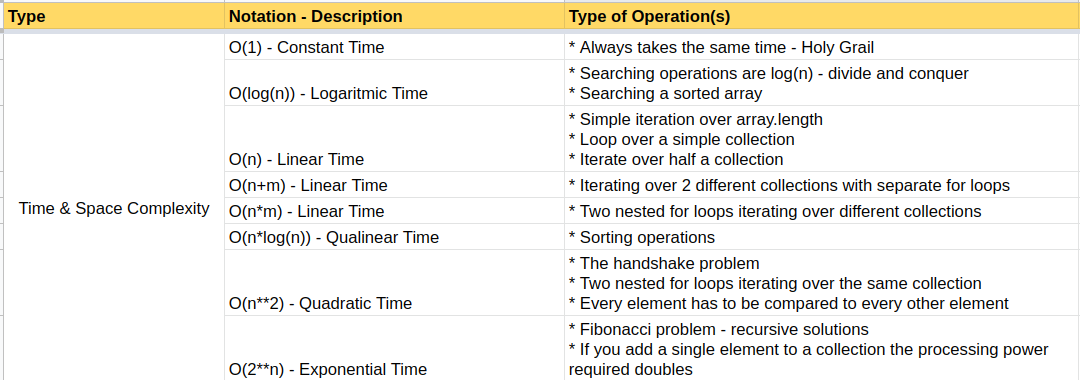Back-end Engineering Articles

I write and talk about backend stuff like Ruby, Ruby On Rails, Databases, Testing, Architecture / Infrastructure / System Design, Cloud, DevOps, Backgroud Jobs, and more...

@daniel_moralesp

2019-07-05

## Introduction to Big O Notation

Now it's time to touch on this important topic in computer science. But to understand these concepts, you've to know about Time and Space Complexity and the basics of memory. Once you have that knowledge, we're ready to start.

# Big O Notation

It is a mathematical notation that describes the limiting behavior of a function when the argument tends towards a particular value or infinity. For example, in computer science, big O notation is used to classify algorithms according to how their run time or space requirements grow as the input size grows.

In simple terms, we can use the big O notation to measure how good an algorithm is based on the time it takes to execute (in seconds) and the space (in memory) that needs to be stored. It's not the same if you have an array of 10 elements and you need to iterate over it than if you have the same array but with 100.000 elements and you also need to iterate over it. Both operations will take different amounts of time and space, and we need a way to measure it. Pay attention here because we're talking about iterating through a simple array of numbers, not about other kinds of operations like ordering from ascending to descending (which actually can take a different amount of time and space).

# N

So, if we have an array of 10 or 100.000 numbers, that total of elements can be denoted as the "N" character. This means that if you have just 1 element in a given array, it doesn't matter the operation itself (iteration, searching, or other) because it will execute almost instantly. But if we increment the "N" to 100.000, it completely changes the time and space that it takes to perform the operation (iteration, searching, or other)

# O(1) - Constant Time

First algorithm

```2.6.8 :016 > array = [1, 2, 3, 4]
=> [1, 2, 3, 4]
2.6.8 :017 > operation_1 = array * 10
=> 10 ```

This operation is a simple creation of an array of 4 elements, and then we select the first position of the array, and we multiply it by 10

This example will always be executed in constant time. So it doesn't matter the number of the elements of the array. Why is that? Because we're just selecting the first element of the array, and then we multiplied by 10. So it doesn't matter the number of elements in the array. This is the Holy Grail, which happens in cases similar to the mentioned one.# O(n) - Linear Time

Second algorithm

```2.6.8 :018 > operation_2 = array.each {|a| puts a}
1
2
3
4
=> [1, 2, 3, 4] ```

In this operation, we used the same array created before, iterated through each array element, and then printed it out.

This example will always be executed in "linear time." As we mentioned above, the "n" is the number of elements of the array (in our example). So if we have 4 elements, it will take 0(4) time and space complexity, and if we have 100.000 elements, it will take 0(100000) time and space. So I think you got it; it's a matter of the size of the array and the operation itself: a simple iteration. The most common use cases here are:

* Simple iteration over the array.length
* Loop over a simple collection of elements
* Iterate over half a collection of elementsThird algorithm

```2.6.8 :019 > operation_3 = array.each do |a1|
2.6.8 :020 >     array.each do |a2|
2.6.8 :021 >       puts a1 * a2
2.6.8 :022?>     end
2.6.8 :023?>   end
1
2
3
4
2
4
6
8
3
6
9
12
4
8
12
16
=> [1, 2, 3, 4] ```

And the last algorithm goes 2 times through the same array of 4 elements and multiplies each element of each iteration.

As we said before, we now have just 4 elements inside the array, but if we increase that number of elements to be 100.000 or even 1 million elements, the time and space will change dramatically.

This example will always be executed in "quadratic time." Because we're iterating two times the same array, we have to square the "n" elements of the array. If we have 4 elements, it will take 0(4**2) time and space complexity, and if we have 100.000 elements, it will take 0(100000**2) time and space. Here is where the algorithm takes much more time and space to be executed. The most common use cases are:

* The handshake problem
* Two nested for loops iterating over the same collection
* Every element has to be compared to every other element# Other Notations Cheatsheet

There are undoubtedly many more notations than mentioned before. Take time to try to understand the other ones, and you can find more on the following resources:

However, I want to share my simple cheatsheet about big O notations with you. These are just the most common ones, so please refer to the links shared above if you need something more profound.# Worst Case Scenarios

If we have an array of 100 elements in ascending order and need to look for a given number, we can use a searching algorithm (later, we'll see the code). What will happen if the number you want to search inside the array is the number 2? It will take just 2 iterations; we call it the best-case scenario. Now you can guess. What will happen if you need to look for the number 99? It will take 99 iterations to search that number. That's near the worst-case scenario. And what will happen if you want to explore the number 50? That's the average-case scenario. So, our coding results needs to be explained as worst-case scenarios (sometimes as average-case scenarios)

Note: this example is merely explanatory, and it doesn't mean that a searching algorithm works precisely like that.

Big O notation is usually understood to describe the worst-case scenario complexity algorithm, even though the worst-case complexity might differ from the average-case complexity. For example, some sporting algorithms have different time complexities depending on the layout of elements in their input array. Thus, when describing the time complexity of an algorithm, it can sometimes be helpful to specify whether the time complexity refers to the average case or the worst case.

In our next blog post, we'll see the algorithms that operate in a logarithmic time and space because they have something special, and we need to learn about it.

I hope you learned a lot from this post.